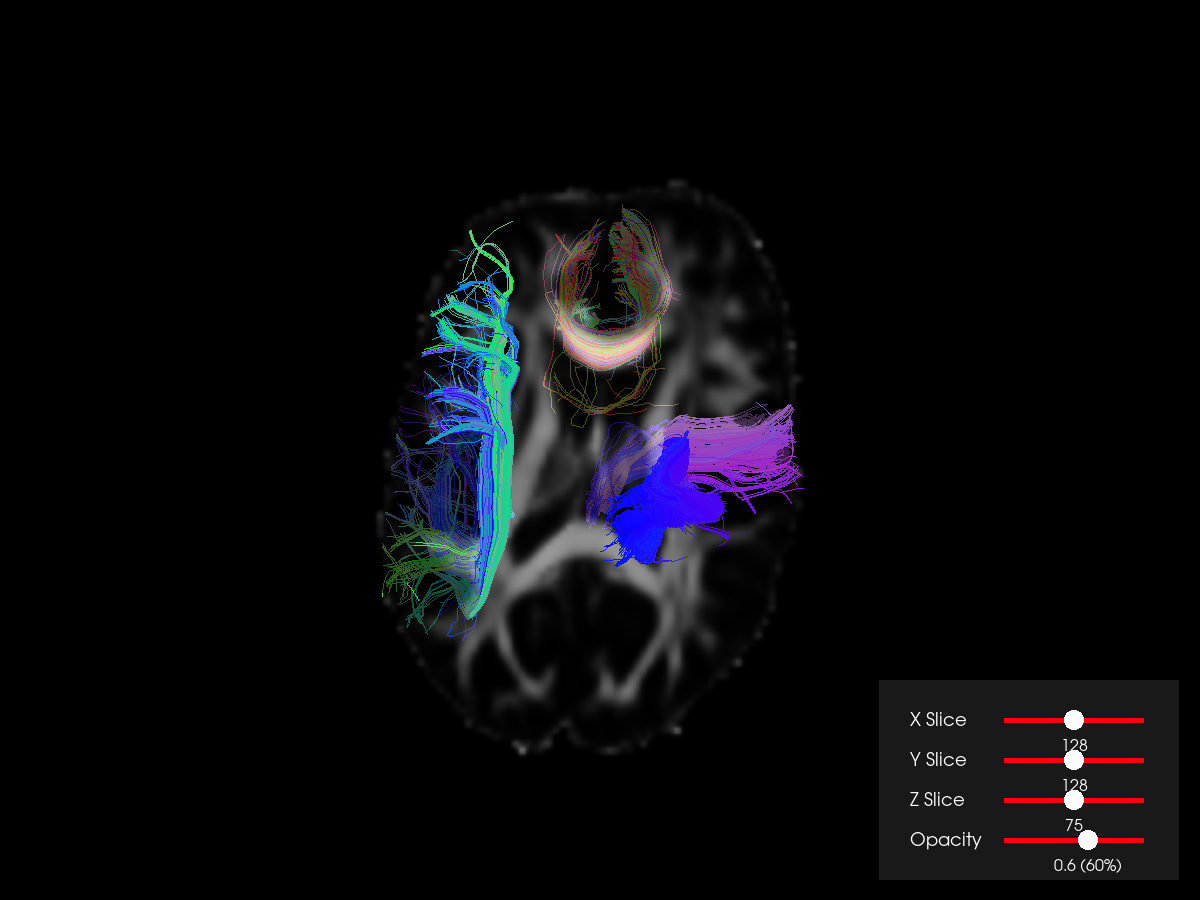In DIPY we created a thin interface to access many of the capabilities available in the Visualization Toolkit framework (VTK) but tailored to the needs of structural and diffusion imaging. Initially the 3D visualization module was named `fvtk`, meaning functions using vtk. This is still available for backwards compatibility but now there is a more comprehensive way to access the main functions using the following modules.

```import numpy as np
from fury import actor, window, ui
```

In `window` we have all the objects that connect what needs to be rendered to the display or the disk e.g., for saving screenshots. So, there you will find key objects and functions like the `Scene` class which holds and provides access to all the actors and the `show` function which displays what is in the scene on a window. Also, this module provides access to functions for opening/saving dialogs and printing screenshots (see `snapshot`).

In the `actor` module we can find all the different primitives e.g., streamtubes, lines, image slices, etc.

In the `ui` module we have some other objects which allow to add buttons and sliders and these interact both with windows and actors. Because of this they need input from the operating system so they can process events.

Let’s get started. In this tutorial, we will visualize some bundles together with FA or T1. We will be able to change the slices using a `LineSlider2D` widget.

First we need to fetch and load some datasets.

```from dipy.tracking.streamline import Streamlines

fetch_bundles_2_subjects()
```

Out:

```Data size is approximately 234MB
Dataset is already in place. If you want to fetch it again please first remove the folder /Users/koudoro/.dipy/exp_bundles_and_maps
```

The following function outputs a dictionary with the required bundles e.g. `af left` (left arcuate fasciculus) and maps, e.g. FA for a specific subject.

```res = read_bundles_2_subjects('subj_1', ['t1', 'fa'],
['af.left', 'cst.right', 'cc_1'])
```

We will use 3 bundles, FA and the affine transformation that brings the voxel coordinates to world coordinates (RAS 1mm).

```streamlines = Streamlines(res['af.left'])
streamlines.extend(res['cst.right'])
streamlines.extend(res['cc_1'])

data = res['fa']
shape = data.shape
affine = res['affine']
```

With our current design it is easy to decide in which space you want the streamlines and slices to appear. The default we have here is to appear in world coordinates (RAS 1mm).

```world_coords = True
```

If we want to see the objects in native space we need to make sure that all objects which are currently in world coordinates are transformed back to native space using the inverse of the affine.

```if not world_coords:
from dipy.tracking.streamline import transform_streamlines
streamlines = transform_streamlines(streamlines, np.linalg.inv(affine))
```

Now we create, a `Scene` object and add the streamlines using the `line` function and an image plane using the `slice` function.

```scene = window.Scene()
stream_actor = actor.line(streamlines)

if not world_coords:
image_actor_z = actor.slicer(data, affine=np.eye(4))
else:
image_actor_z = actor.slicer(data, affine)
```

We can also change also the opacity of the slicer.

```slicer_opacity = 0.6
image_actor_z.opacity(slicer_opacity)
```

We can add additonal slicers by copying the original and adjusting the `display_extent`.

```image_actor_x = image_actor_z.copy()
x_midpoint = int(np.round(shape / 2))
image_actor_x.display_extent(x_midpoint,
x_midpoint, 0,
shape - 1,
0,
shape - 1)

image_actor_y = image_actor_z.copy()
y_midpoint = int(np.round(shape / 2))
image_actor_y.display_extent(0,
shape - 1,
y_midpoint,
y_midpoint,
0,
shape - 1)
```

Connect the actors with the Scene.

```scene.add(stream_actor)
```

Now we would like to change the position of each `image_actor` using a slider. The sliders are widgets which require access to different areas of the visualization pipeline and therefore we don’t recommend using them with `show`. The more appropriate way is to use them with the `ShowManager` object which allows accessing the pipeline in different areas. Here is how:

```show_m = window.ShowManager(scene, size=(1200, 900))
show_m.initialize()
```

After we have initialized the `ShowManager` we can go ahead and create sliders to move the slices and change their opacity.

```line_slider_z = ui.LineSlider2D(min_value=0,
max_value=shape - 1,
initial_value=shape / 2,
text_template="{value:.0f}",
length=140)

line_slider_x = ui.LineSlider2D(min_value=0,
max_value=shape - 1,
initial_value=shape / 2,
text_template="{value:.0f}",
length=140)

line_slider_y = ui.LineSlider2D(min_value=0,
max_value=shape - 1,
initial_value=shape / 2,
text_template="{value:.0f}",
length=140)

opacity_slider = ui.LineSlider2D(min_value=0.0,
max_value=1.0,
initial_value=slicer_opacity,
length=140)
```

Now we will write callbacks for the sliders and register them.

```def change_slice_z(slider):
z = int(np.round(slider.value))
image_actor_z.display_extent(0, shape - 1, 0, shape - 1, z, z)

def change_slice_x(slider):
x = int(np.round(slider.value))
image_actor_x.display_extent(x, x, 0, shape - 1, 0, shape - 1)

def change_slice_y(slider):
y = int(np.round(slider.value))
image_actor_y.display_extent(0, shape - 1, y, y, 0, shape - 1)

def change_opacity(slider):
slicer_opacity = slider.value
image_actor_z.opacity(slicer_opacity)
image_actor_x.opacity(slicer_opacity)
image_actor_y.opacity(slicer_opacity)

line_slider_z.on_change = change_slice_z
line_slider_x.on_change = change_slice_x
line_slider_y.on_change = change_slice_y
opacity_slider.on_change = change_opacity
```

We’ll also create text labels to identify the sliders.

```def build_label(text):
label = ui.TextBlock2D()
label.message = text
label.font_size = 18
label.font_family = 'Arial'
label.justification = 'left'
label.bold = False
label.italic = False
label.background_color = (0, 0, 0)
label.color = (1, 1, 1)

return label

line_slider_label_z = build_label(text="Z Slice")
line_slider_label_x = build_label(text="X Slice")
line_slider_label_y = build_label(text="Y Slice")
opacity_slider_label = build_label(text="Opacity")
```

Now we will create a `panel` to contain the sliders and labels.

```panel = ui.Panel2D(size=(300, 200),
color=(1, 1, 1),
opacity=0.1,
align="right")
panel.center = (1030, 120)

```

Then, we can render all the widgets and everything else in the screen and start the interaction using `show_m.start()`.

However, if you change the window size, the panel will not update its position properly. The solution to this issue is to update the position of the panel using its `re_align` method every time the window size changes.

```size = scene.GetSize()

def win_callback(obj, _event):
global size
if size != obj.GetSize():
size_old = size
size = obj.GetSize()
size_change = [size - size_old, 0]
panel.re_align(size_change)

show_m.initialize()
```

Finally, please set the following variable to `True` to interact with the datasets in 3D.

```interactive = False

scene.zoom(1.5)
scene.reset_clipping_range()

if interactive:

show_m.render()
show_m.start()

else:

window.record(scene, out_path='bundles_and_3_slices.png', size=(1200, 900),
reset_camera=False)

del show_m
```Total running time of the script: ( 0 minutes 3.934 seconds)

Gallery generated by Sphinx-Gallery